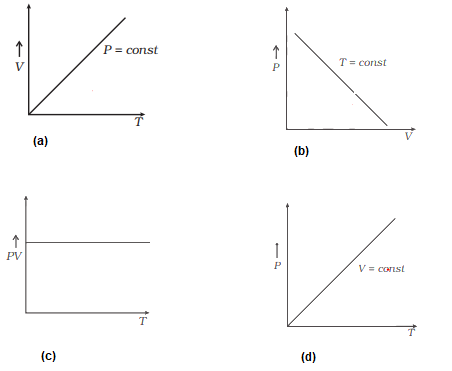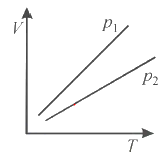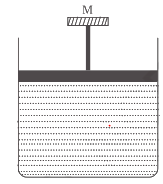# Ideal Gas Laws Problems

A box Of interior Volume $V_1$ has a heavy airtight hinged lid of mass M and area A.The box contains n1 moles of gas at Temperature T0.The box is inside a chamber which also contains additional n2 moles of the same gas at the same temperature..The gas in the chamber occupies the volume V2.

Question 1
find the pressure of the gas in the box
a. n1RT0/V1
b. n2RT0/V2
c. (n1+n2)RT/V1+V2
d. none of the above.

Question 2
find the pressure of the gas in the chamber
a. n1RT0/V1
b. n2RT0/V2
c. (n1+n2)RT/V1+V2
d. none of the above.

Question 3
Initially the hinged lid is closed.Then which of these expression is true
a.n1RT0/V1 -n2RT0/V2 <=Mg/A
b. n1RT0/V1 -n2RT0/V2 => Mg/A
c. n2RT0/V2 -n1RT0/V1 => Mg/A
d. n2RT0/V2 -n1RT0/V1 <= Mg/A

Question 4
If the whole system is heated,at what temperature T will the gas pressure lift the hinged lid
a. T=(Mg/RA)(n1/V1 -n2/V2)
b. T=(Mg/RA)(n2/V2 -n1/V1)
c. T=(Mg/RA)(n1/V1 +n2/V2)
d. None of the above

## Multiple choice questions with one or more answer

Question 5
In the vander waals equation
(P+a/V2)(V-b)=nRT

a. a and b are the correction for the cohesive forces
b. a and b are the correction for the volume occupied by the molecules.
c. a is the correction for the volume occupied by the molecules and b is the correction for the cohesive forces
d. a is the correction for the cohesive forces and b is the correction for the volume occupied by the molecules

An gas obeys Vander waals equation
(P+a/V2)(V-b)=nRT

Question 6
The dimensional formula for constant a is
a. ML5T-2
b. ML5T-1
c. ML-1T-1
d. M0L-0T0

Question 7
Find the work done by this gas when gas expand from V to 2V at constant temperature T
a.nRTloge(2V-b/V-b) -a/V
b.nRTloge(2V-b/V-b) +a/V
c. nRTloge(2V-b/V-b)
d. none of the above

Assertion and Reason
a. Statement I is true ,statement II is true ,statement II is correct explanation for statement I
b. Statement I is true ,statement II is true ,statement II is not a correct explanation for statement I
c. Statement I is true,Statement II is false
d. Statement I is False,Statement II is True
Question 8
STATEMENT 1:Real Gases behave like ideal gas at high temperature
STATEMENT 2:Intermolecular forces are negligible at high temperature

Question 9
STATEMENT 1:Equation of state for a real gas is (P+a/V2)(V-b)=nRT
STATEMENT 2: Molecular attraction is not negligible and the size of molecules are not negligible in comparison to average separation between them

Question 10
STATEMENT 1:The pressure exerted by a gas is the same irrespective of the size of the molecules
STATEMENT II:Molecular collisions will invariably change the velocities of individual molecules but the number of the molecules having any particular velocity will remain change

Question 11
STATEMENT 1: mean kinetic energy of molecule are same for all gases at the same temperature
STATEMENT 2: Heavy molecules will moves slower than light molecules at the same temperature

## Multiple choice questions with one or more answer

Question 12
which of the following pairs are correctly matched
Law of equipartition of energy :Boltzmann
Law of distribution of speed :Maxwell
Law of liquefaction of gases:Joule Kelvin
a. 1
b. 2
c. 3
d. none of the above

Question 13
Which of the following statement is correct about ideal gas
a. In Isothermal Process PV=constant
b. In adiabatic process $PV^{\gamma}=constant$
c. In isothermal process,root mean square velocity does not change
d. none of the above

A vessel contains two non-reactive gases : neon (monatomic) and oxygen (diatomic). The ratio of their partial pressures is 3:2. Atomic mass of Ne = 20.2 u, molecular mass of O2 = 32.0 u.
Question 14
Estimate the ratio of number of molecules
a. 1:2
b. 2:3
c. 3:2
d. 9:4

Question 15
find the ratio of mass density of neon and oxygen in the vessel
a. .946:1
b. .745:1
c. .56:16
d. .80:1

Question 16
Boyle's law is applicable for an
(b) isothermal process.
(c) isobaric process.
(d) Isochoric process.

Question 17
An inflated rubber balloon contains one mole of an ideal gas, has a pressure p, volume V and temperature T. If the temperature rises to 1.1 T, and the volume is increased to 1.05 V, the final pressure will be
(a) 1.1 p
(b) p
(c) less than p
(d) between p and 1.1p

Question 18
Which of the following diagrams depicts ideal gas behaviour?Question 19
Volume versus temperature graphs for a given mass of an ideal gas are shown in below at two different values of constant pressure. What can be inferred about relation between $p_1$ & $p_2$?a. $p_1 =p_2$
b. $p_1 > p_2$
c. $p_1 < p_2$
d. data is insufficient

Question 20
A cylinder containing an ideal gas is in vertical position and has a piston of mass M that is able to move up or down without friction
If the temperature is increased,(a) both p and V of the gas will change.
(b) only p will increase according to Charle's law.
(c) V will change but not p.
(d) p will change but not V.

## Subjective Problem

Question 21
One mole of ideal gas undergoes a process
P=P0/(1+(V/V0)2)
Where P0 and V0 are constant. Find the temperature of gas when V=V0

Question 22.
Calculate the variation of atmospheric pressure with elevation of the earth atmosphere. Considering the temperature to be uniform throughout (which is not the actual case)